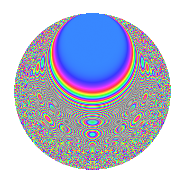# Properties

 Label 2805.2.gLevel 2805 Weight 2 Character orbit g Rep. character $$\chi_{2805}(1189,\cdot)$$ Character field $$\Q$$ Dimension 184 Sturm bound 864

# Related objects

## Defining parameters

 Level: $$N$$ = $$2805 = 3 \cdot 5 \cdot 11 \cdot 17$$ Weight: $$k$$ = $$2$$ Character orbit: $$[\chi]$$ = 2805.g (of order $$2$$ and degree $$1$$) Character conductor: $$\operatorname{cond}(\chi)$$ = $$85$$ Character field: $$\Q$$ Sturm bound: $$864$$

## Dimensions

The following table gives the dimensions of various subspaces of $$M_{2}(2805, [\chi])$$.

Total New Old
Modular forms 440 184 256
Cusp forms 424 184 240
Eisenstein series 16 0 16

## Trace form

 $$184q - 200q^{4} + 184q^{9} + O(q^{10})$$ $$184q - 200q^{4} + 184q^{9} + 232q^{16} + 16q^{19} - 24q^{25} - 48q^{26} + 32q^{30} + 24q^{34} + 16q^{35} - 200q^{36} + 200q^{49} - 16q^{50} + 8q^{51} + 16q^{59} - 296q^{64} - 16q^{66} - 32q^{70} - 16q^{76} + 184q^{81} + 64q^{84} - 24q^{85} - 64q^{86} - 144q^{94} + O(q^{100})$$

## Decomposition of $$S_{2}^{\mathrm{new}}(2805, [\chi])$$ into newform subspaces

The newforms in this space have not yet been added to the LMFDB.

## Decomposition of $$S_{2}^{\mathrm{old}}(2805, [\chi])$$ into lower level spaces

$$S_{2}^{\mathrm{old}}(2805, [\chi]) \cong$$ $$S_{2}^{\mathrm{new}}(85, [\chi])$$$$^{\oplus 4}$$$$\oplus$$$$S_{2}^{\mathrm{new}}(255, [\chi])$$$$^{\oplus 2}$$$$\oplus$$$$S_{2}^{\mathrm{new}}(935, [\chi])$$$$^{\oplus 2}$$